Mathematics

# Weight, Mass, and Density Unit Conversions

999 views

 1 Introduction 2 Definitions 3 Unit Conversion for Mass 4 Unit Conversion for Weight 5 Unit Conversion for Density 6 Summary 7 FAQs

## Introduction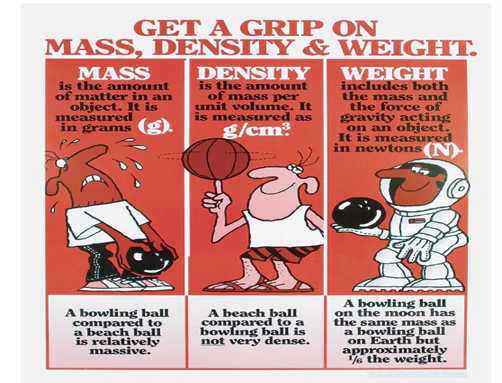Source: cosmicframe.wordpress

Weight, Mass, and Density is quite an intriguing topic. They all sound the same, isn’t it? Here is the surprising element: though Weight, mass, and density depend on one another they are different from each other. To make a clear understanding, the above image is explained below.

### Weight, Mass, and Density Unit Conversions-PDF

Learn about the Conversion of Units of Weight, Mass, and Density. g to kg, kg to lbs, lbs to kg, etc. with examples. Here is a downloadable PDF to explore more.

## Definitions

### Mass:

Every object is made up of atoms; mass is approximately the number of atoms in an object and also the measure of the object’s resistance towards the gravity. Mass remains the same irrespective of the place.

The SI unit of Mass is: Kilogram

 MASS Mass on Earth Mass on Moon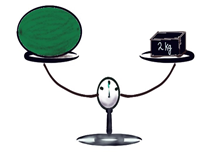### Weight:

Weight depends on the force of the gravity on any object. Unlike mass, weight keeps changing it’s number based on the place.

The SI Unit for weight is: Newton.

Weight is calculated using the formula:

W= mg

Whereas,

W = weight

M = mass

g= Gravitational force on the object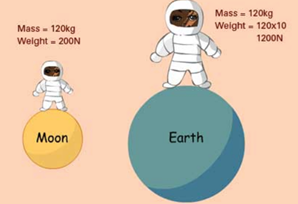source:https://eschooltoday.com/science/forces/what-is-mass.html

### Density

The density of a material depends on the volume of the material; it depends on the dispersion of the atoms that occupy per unit volume of the space.

Formula:

$$\rho=\frac{m}{v}$$

Whereas,

\begin{align}\rho&=\text{density}\\m &= \text{mass}\\V &= \text{Volume}\end{align}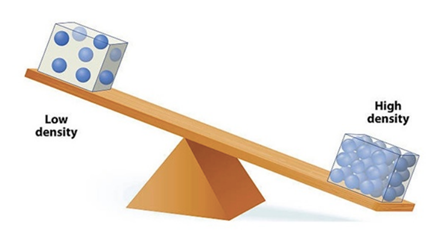source:https://www.quora.com/Does-an-object-having-greater-mass-have-greater-weight-and-greater-density

## Unit Conversion for Mass

### Unit Converter

 mg to g: Gram(g) is used as a unit of the weight of an object 1 mg = 0.001gm; is obtained by dividing the mass value by 1000 g to kg Kg is the base unit of mass in the current system and has the unit kg 1 g = 0.001 kg; is obtained by dividing the mass value by 1000 Kg to g Kg is the base unit of mass in the current system and has the unit kg 1 Kg = 1000 g; is obtained by multiplying the mass value by 1000 g to mg One of the unit of measurement of mass in the current system and is 1/1000th of gram 1 g = 1000 mg Kg to mg As we know by now that 1 kg is 1000 gms and 1gms is 1000 mgms; converting them in terms of kg would be product of gms and mgms 1 kg = 1000 × 1000 = 1000000 mgms mg to g Since mg is 1/1000th of gm the mass; the formula can be written as; 1 mg = 0.001 gms lbs to oz lbs represents the unit of pound; which is the imperial mass unit. 1lb = 16oz oz to lbs oz is the abbreviation of ounces; is also used as a measurement of weight; which is 1/16th the value of pounds 1 oz = 0.0625 lbs Kg to ton Ton is the heaviest unit of weight 1 Kg = 1/1000th ton Ton to kg Ton is measured as 1000 times the weight of an object in kgs 1 ton = 1000 kgs

 Examples

 80 kg to lbs 1 Kg = 2.2 lbs For 80 kgs = 80 $$×$$ 2.2 = 176 lbs 150 lbs to kg 1 lb = 1/2.2 kgs For 150lbs = 150/2.2 kgs = 68.18 kgs 50kg to lbs 1 kg = 2.2 lbs For 50 kgs = 50 $$×$$ 2.2 = 110 lbs 23 kg to lbs 1 kg = 2.2 lbs For 23 kgs = 23 $$×$$ 2.2 = 50.6 lbs 160 lbs to kg 1 lb = 1/2.2 kgs For 160lbs = 160/2.2 kgs = 72.7272 kgs 75 kg to lbs 1 kg = 2.2 lbs For 75 kgs = 75 $$×$$2.2 = 165 lbs 200 lbs to kg 1 lb = 1/2.2 kgs For 200lbs =200/2.2 kgs =90.91 kgs 130 lbs to kg 1 lb = 1/2.2 kgs For 130lbs = 130/2.2 kgs = 59.091 kgs 180 lbs to kg 1 lb = 1/2.2 kgs For 180lbs = 180/2.2 kgs = 68.18 150 kg to lbs 1 kg = 2.2 lbs For 150 kgs = 150 $$×$$ 2.2 = 110 lbs 145 lbs to kg 1 lb = 1/2.2 kgs For 145lbs = 145/2.2 kgs = 65.909 kgs 120kg to lbs 1 kg = 2.2 lbs For 120 kgs = 120 $$×$$ 2.2 = 110 lbs

## Unit Conversion for Weight

### Unit Converter

 kg to newtons: By using the Newton’s second law of motion, Kg and newton can be relatively expressed as: $$1N = Kg \times m/s^2$$ Whereas, N = Newton Kg= Kilogram m= meter(distance travelled) S = time duration in seconds Therefore, a force needed to accelerate 1 kg of mass at the rate of 1m/s2  is always equal to 1N lbf to n: lbf ; Pound of force; it’s the unit of force that is acted on by one pound of mass in a standard gravitational field. 1 lbf ≅ 4.44822 N Kn to N: Kn is nothing but KiloNewton; 1Kn = 1000 N N to lbf: lbf ; Pound of force; it’s the unit of force that is acted on by one pound of mass in a standard gravitational field. 1N ≅ 0.224809 lbf lbs to newtons: lbs is the imperial system of unit of mass 1lbs ≅ 4.44822 N gf to Kgf: Kgf is the amount of force acted on one kilogram of mass 1 gf = 0.001 Kgf Kgf to gf: Kgf is the amount of force acted on one kilogram of mass 1 Kgf = 1000 gf gf to n: gf is “gram force” force acted on 1 gram of mass in a standard gravitation field 1 gf = 0.00980665 newton

## Unit Conversion for Density

### Unit Converter

 g/cm3 to kg/m3 1kg = 1000 gms 1m = 100 cm Hence,  1g/cm3 = 1000 kg/m3 Density Of Gold Gold is one of the densest metal on earth; it weighs 19.32 gms per cubic centimeter kg/m3 to g/cm3 Since 1kg is 1000gms 1 gm = 1/1000 ∴ 1 kg/m = 1/1000= 0.001 g/cm3 It’s better to convert the values ahead before the calculation g/ml to kg/l 1 gm = 1000 kg 1 ltr = 1000 ml By multiplying the grams by 1000 we can convert the values to kgms Similarly the value from ml can be converted by multiplying the values by 1000 For the above conversion, it’s better to multiply the numerator and the denominator by 1000 The value is 1=1 g/l to kg/m3 The formula goes by dividing mass by volume In this case, 1 cubic meter of a container can hold 1000 litres; 1 g= 1000 kg So the conversion will be 1=1 g/ml to g/l 1l = 1000 ml ∴ by multiplying both numerator and denominator by 1000; ml converts itself to litres whereas 1000 remains Hence 1 g/ml = 1000 mg/dl to g/l 1 mg = 0.001 g 1 dl = 0.1 l ∴ by converting and dividing using the formula mass/volume, we get the answer 0.01 g/l to g/ml 1l = 1000 ml ∴ dividing by 1000; ml converts itself to litres whereas 1000 remains Hence 1 g/l = 0.001 g/ml mg/ml to g/l 1l = 1000 ml ∴ by multiplying both numerator and denominator by 1000; cancelling the like terms we get 1=1 as an answer.

## Summary

To summarise the facts learnt;

Mass is the number of atoms packed in an object; it remains the same irrespective of the force acting on it.

SI unit of mass is Kilogram.

Weight is the force acted on mass pulling towards the ground; it depends on the gravitational force on which the object is placed .

Formula: weight = mass $$×$$ gravity

The SI unit of weight is newton.

Density is the amount of mass that packed inside the volume of any object. Density depends on the volume and the mass;

Formula : density = mass/volume

Written by Santhalakshmi, Cuemath Teacher

## What is density?

Density is the amount of matter inside an object of any volume, regardless of the volume; density can be inversely proportional to an object’s volume.

Formula:

\begin{align}\rho=\frac{m}{v}\end{align}

Whereas,

\begin{align}\rho&=\text{density}\\m &= \text{mass}\\V &= \text{Volume}\end{align}

For Example, A small iron nail may be less in volume but the amount of matter packed inside is more than a large balloon filled with air. In that case, density of the iron nail is more than the density of the balloon.## What is the difference between weight and mass?

In simple words; mass is the quantity of atoms in an object; whereas weight depends on the action of gravity on the quantity of atoms in that object.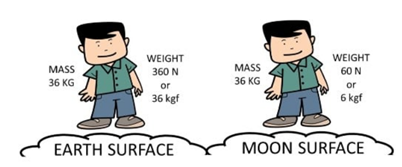source:http://selftution.com/mass-vs-weight/

## What is the density of gold?

Gold is one of the densest metal on earth; it weighs 19.32 grams per cubic centimeter

1 kg = 2.2 lbs

## How many pounds is 23kg?

1 kg = 2.2 lbs

When 1 kg is 2.2 lbs; 23kg would be $$23 × 2.2 = 50.6$$ lbs

## How many kgs in a ton?

There are 1000 kgs in a ton.

Award-winning math curriculum, FREE for a year